# THREE PHASE SYSTEM Introduction Single Phase and Three

• Slides: 96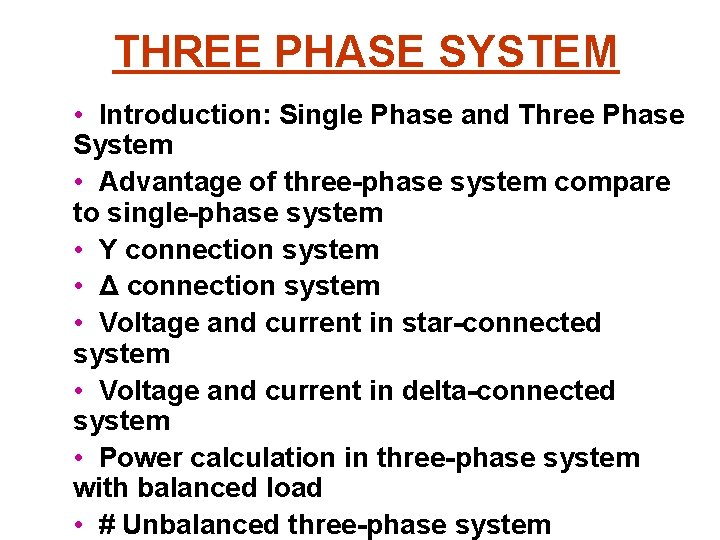THREE PHASE SYSTEM • Introduction: Single Phase and Three Phase System • Advantage of three-phase system compare to single-phase system • Y connection system • Δ connection system • Voltage and current in star-connected system • Voltage and current in delta-connected system • Power calculation in three-phase system with balanced load • # Unbalanced three-phase system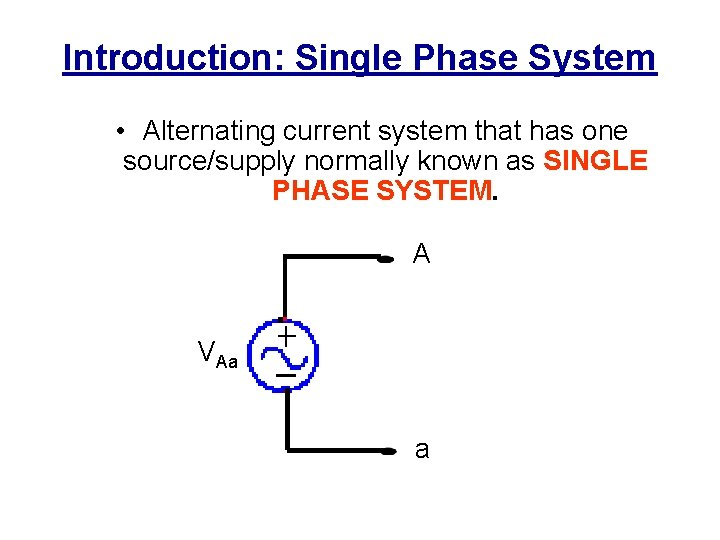Introduction: Single Phase System • Alternating current system that has one source/supply normally known as SINGLE PHASE SYSTEM. A VAa a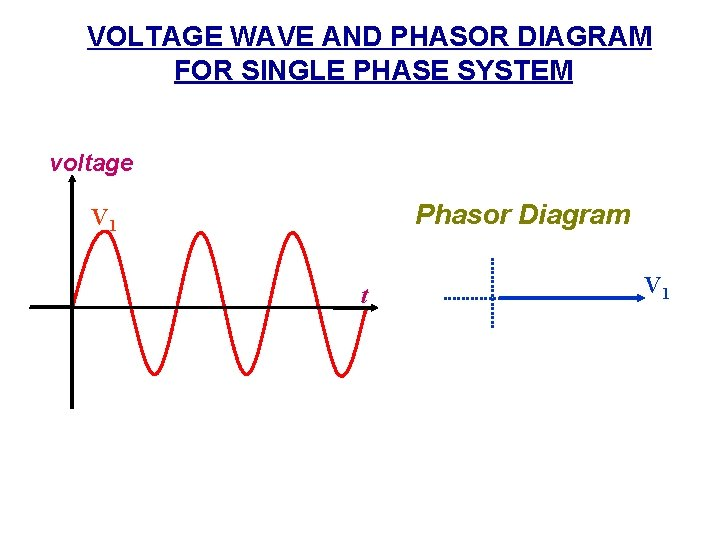VOLTAGE WAVE AND PHASOR DIAGRAM FOR SINGLE PHASE SYSTEM voltage Phasor Diagram V 1 t V 1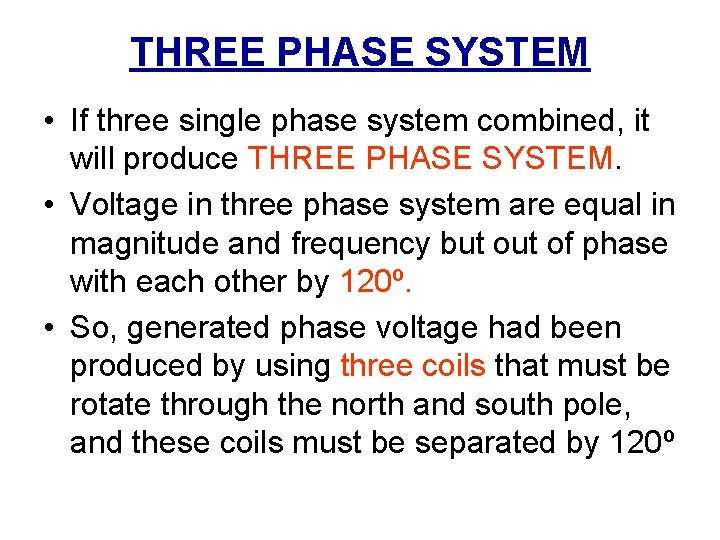THREE PHASE SYSTEM • If three single phase system combined, it will produce THREE PHASE SYSTEM. • Voltage in three phase system are equal in magnitude and frequency but of phase with each other by 120º. • So, generated phase voltage had been produced by using three coils that must be rotate through the north and south pole, and these coils must be separated by 120ºBasic Three-Phase Circuit Three-Phase Line Three. Phase Voltage Source Three. Phase Load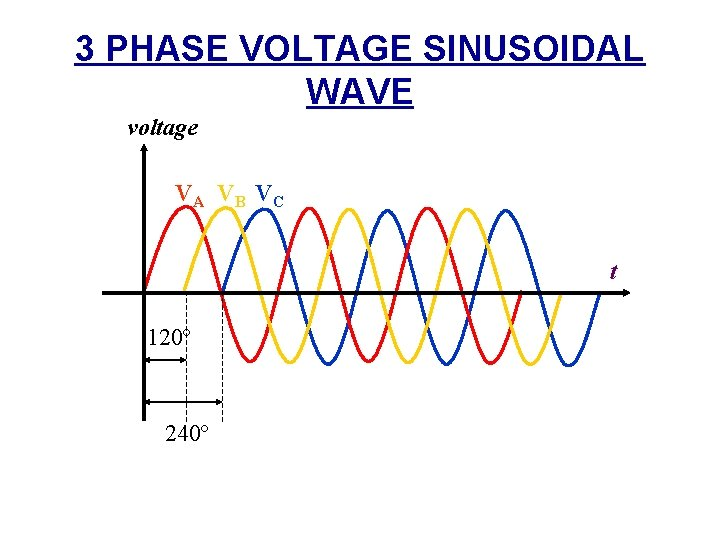3 PHASE VOLTAGE SINUSOIDAL WAVE voltage VA VB VC t 120º 240º• • Normally, we take VA as a reference to VC and VB. So, there are TWO relationship between voltage VA, VB and VC : i. VB lagging VA by 120º and VC leading VA by 120º. This is known as Sequence ABC (positive) ii. VB leading VA by 120º dan VC lagging VA by 120º. This is known as Sequence ACB (negatif)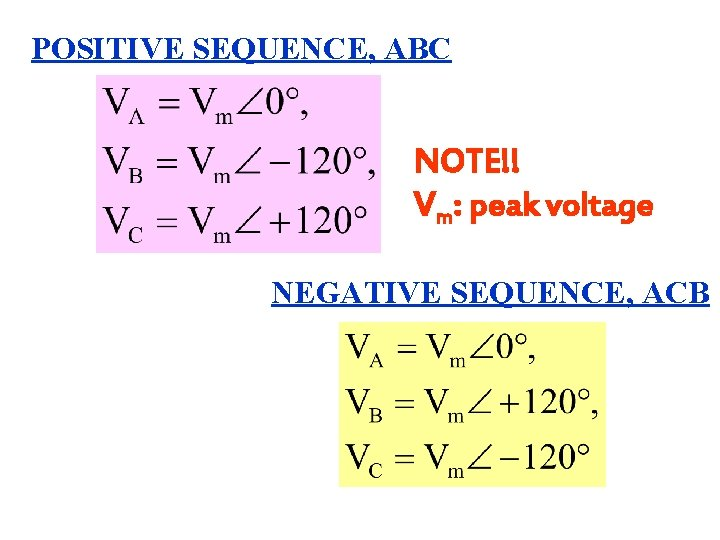POSITIVE SEQUENCE, ABC NOTE!! Vm: peak voltage NEGATIVE SEQUENCE, ACBPOSITIVE SEQUENCE, ABC VC 120º VA 120º VB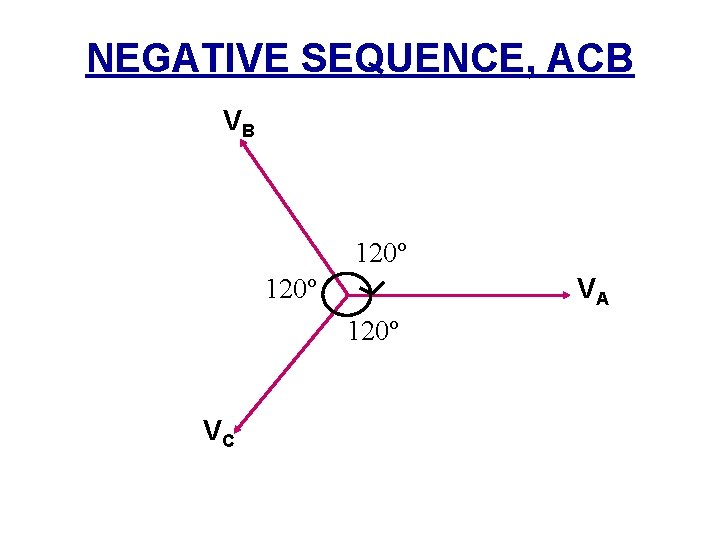NEGATIVE SEQUENCE, ACB VB 120º VA 120º VC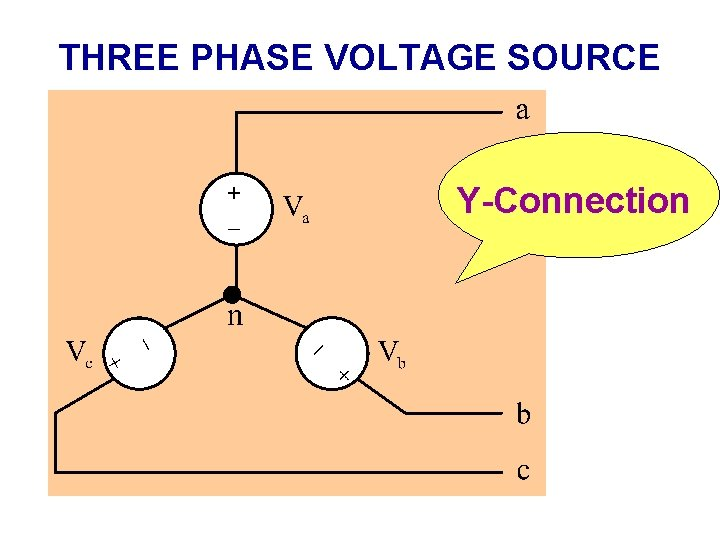THREE PHASE VOLTAGE SOURCE Y-Connection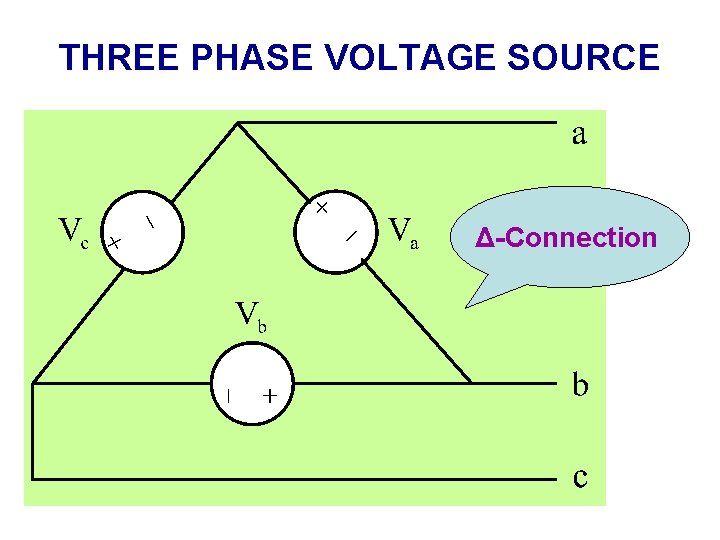THREE PHASE VOLTAGE SOURCE Δ-Connection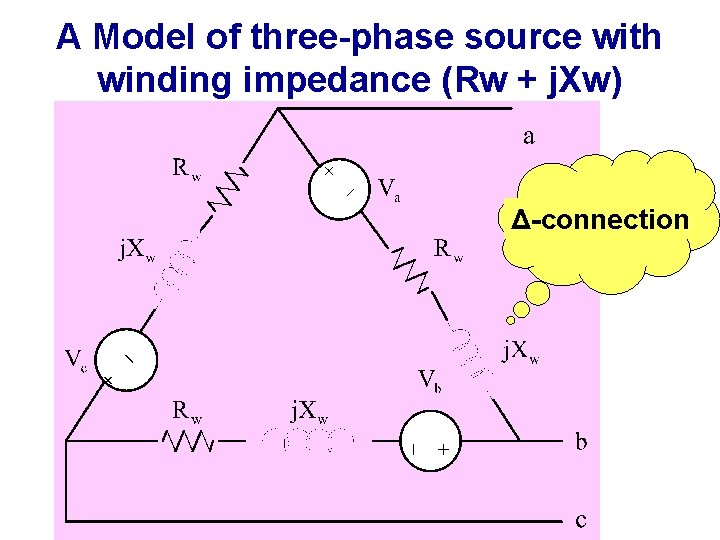A Model of three-phase source with winding impedance (Rw + j. Xw) Δ-connection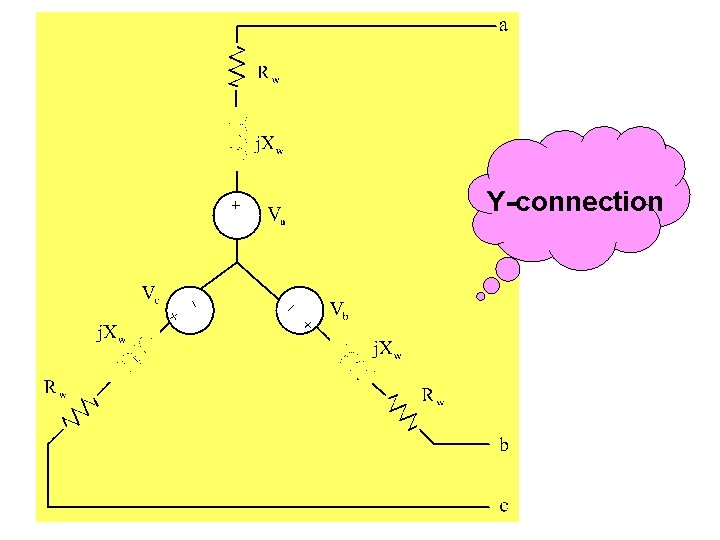Y-connection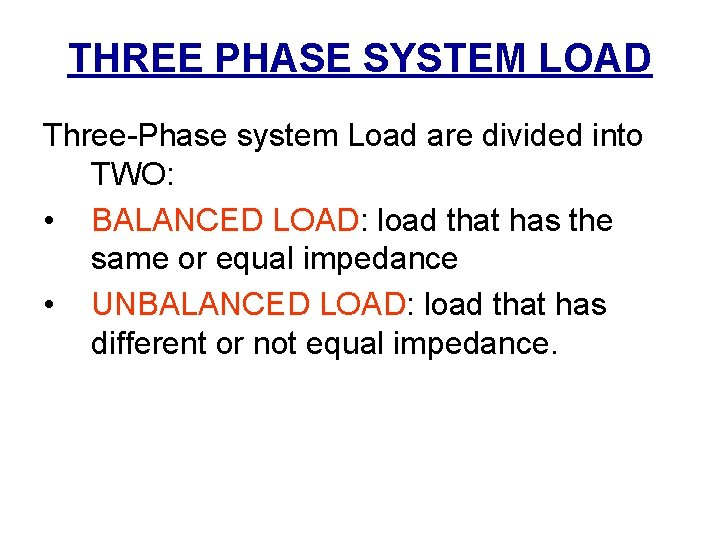Advantages of three-phase system compare to single-phase system • Three-phase transformer is much lighter, better efficiency and more economical compare to single-phase transformer that has same size. • Three-phase motor produce permanent torque while single-phase induction motor produce torque in pulse. • Three-phase motor have power factor and much better efficiency and small size for the same output compare to single-phase motor. • Three-phase motor is self-starter compared to single-phase motor that is not self-starter.THREE PHASE SYSTEM CONNECTION • There are TWO types of connection: i. STAR connection ii. DELTA connection • • Each of this connection is differ in terms of relationship between voltage or current. This connection is connected to both LOAD and SOURCE.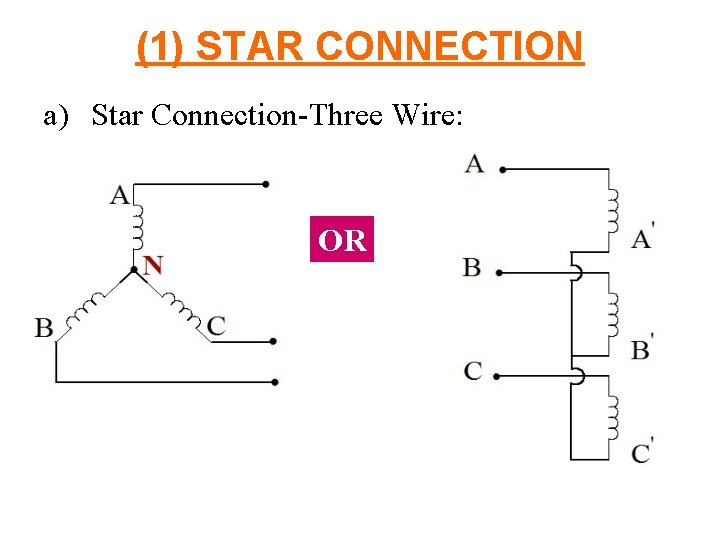(1) STAR CONNECTION a) Star Connection-Three Wire: ORb) Star Connection-Four Wire OR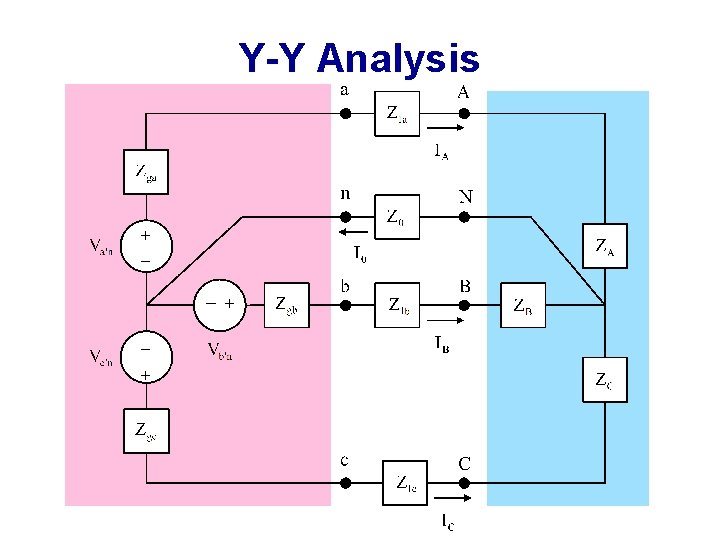Y-Y AnalysisSingle Phase equivalent circuit (a phase as a reference)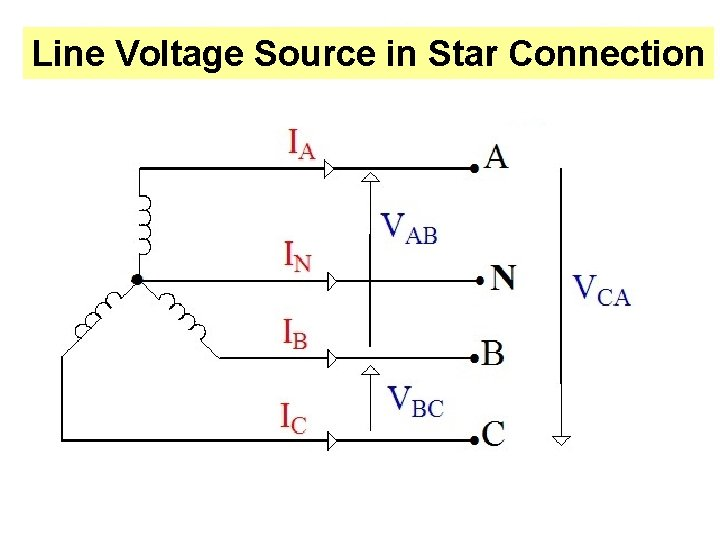Line Voltage Source in Star Connection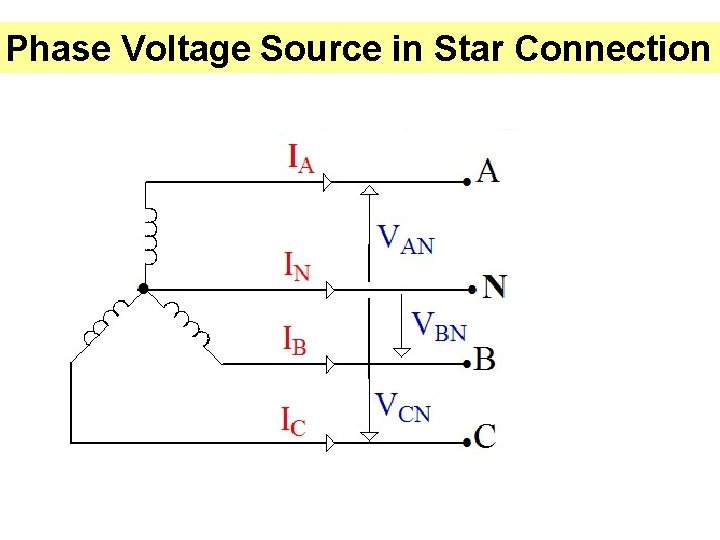Phase Voltage Source in Star Connection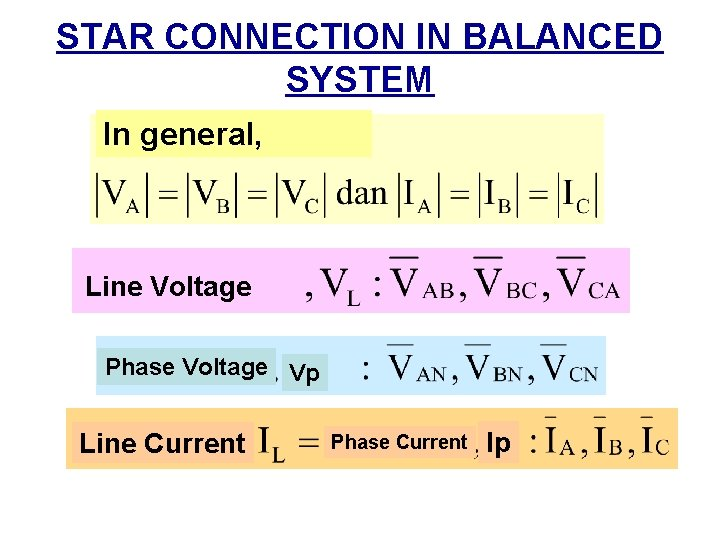STAR CONNECTION IN BALANCED SYSTEM In general, Line Voltage Phase Voltage Vp Line Current Phase Current Ip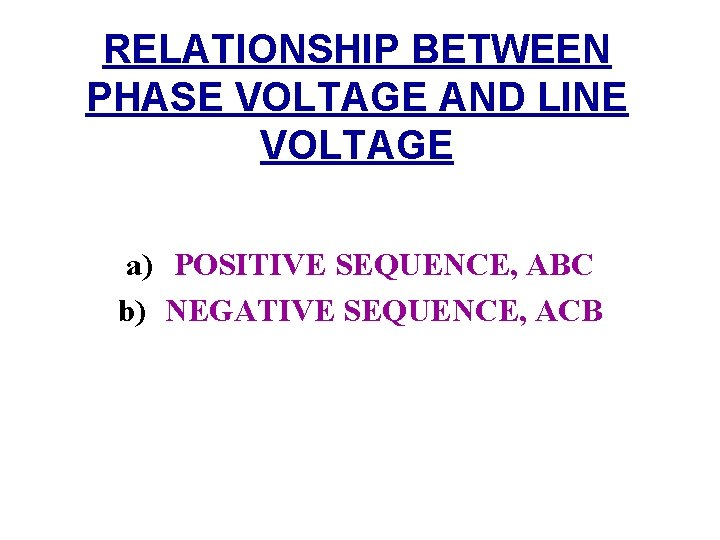RELATIONSHIP BETWEEN PHASE VOLTAGE AND LINE VOLTAGE a) POSITIVE SEQUENCE, ABC b) NEGATIVE SEQUENCE, ACB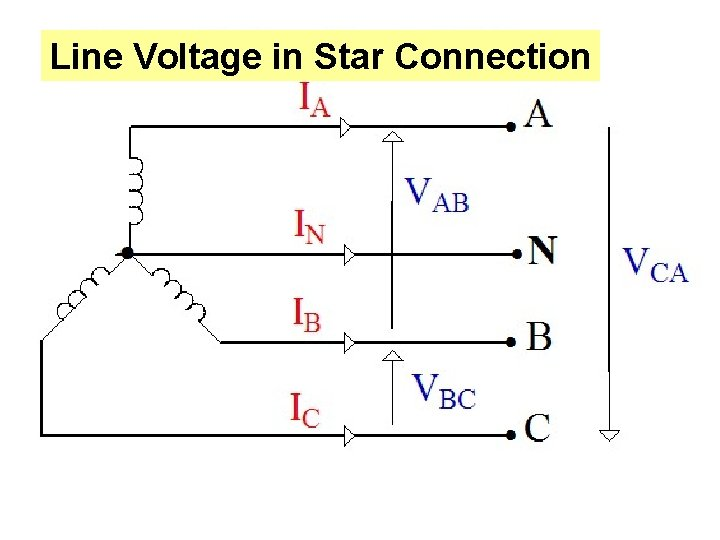Line Voltage in Star Connectiona) POSITIVE SEQUENCE By consider phase voltage VAN as a reference;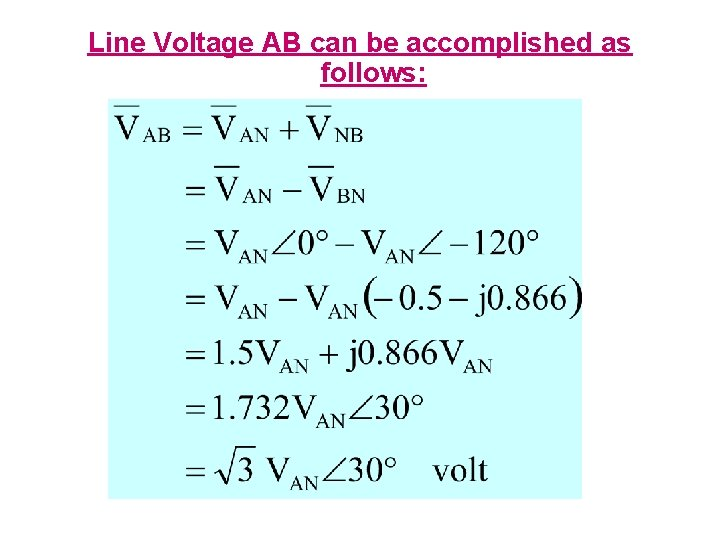Line Voltage AB can be accomplished as follows:Line Voltage BC can be accomplished as follows: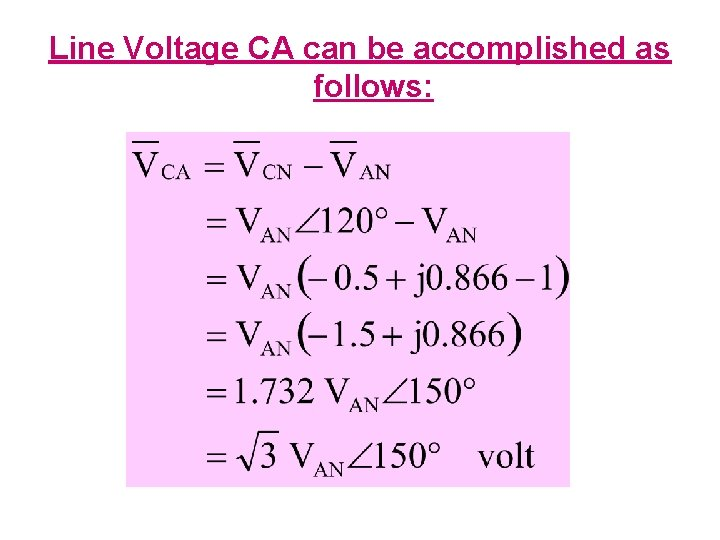Line Voltage CA can be accomplished as follows: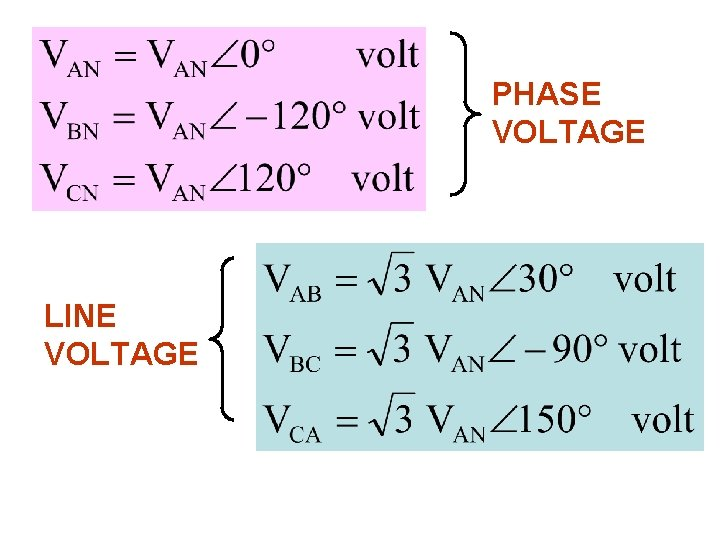PHASE VOLTAGE LINE VOLTAGEPHASOR DIAGRAM VL, VP POSITIVE SEQUENCE VCA VCN VAB 30º 120º 30º VBN VBC 120º VAN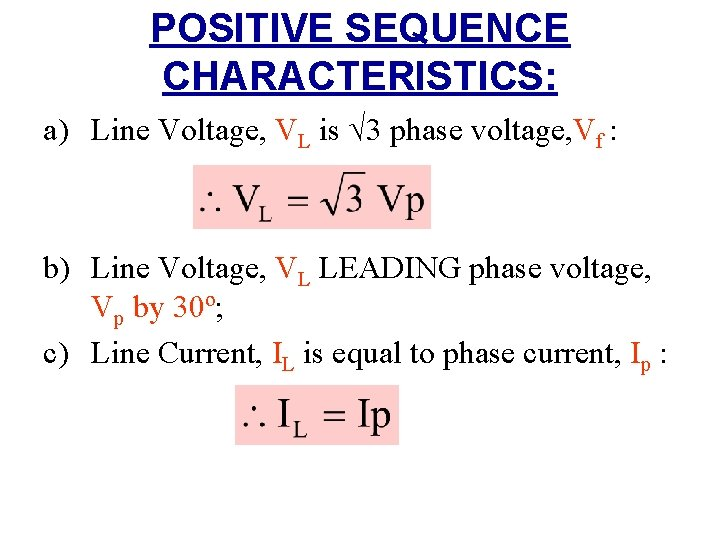POSITIVE SEQUENCE CHARACTERISTICS: a) Line Voltage, VL is √ 3 phase voltage, Vf : b) Line Voltage, VL LEADING phase voltage, Vp by 30º; c) Line Current, IL is equal to phase current, Ip :b) NEGATIVE SEQUENCE By consider the phase voltage VAN as a reference;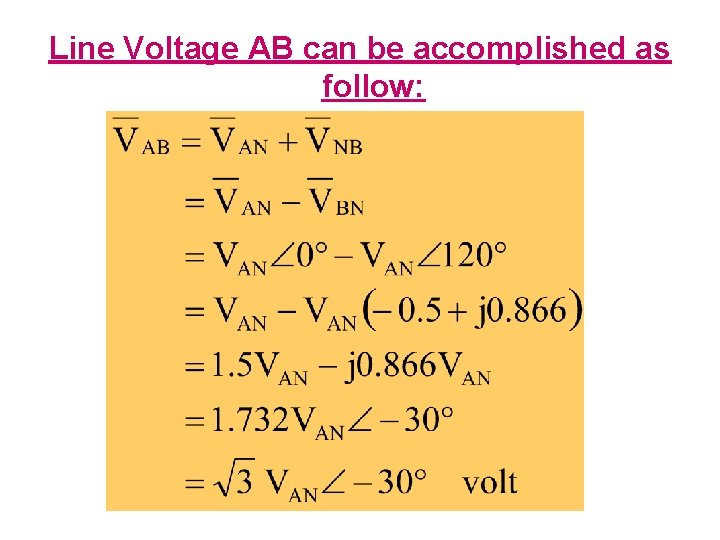Line Voltage AB can be accomplished as follow:Line Voltage BC can be accomplished as follows: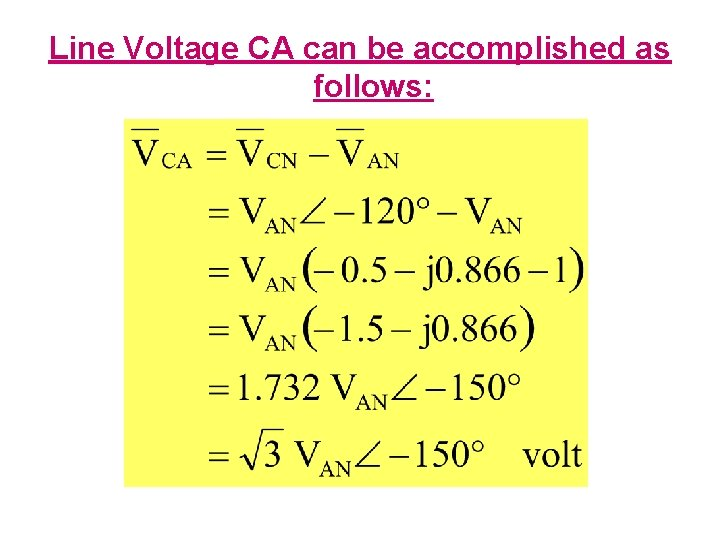Line Voltage CA can be accomplished as follows:PHASE VOLTAGE LINE VOLTAGE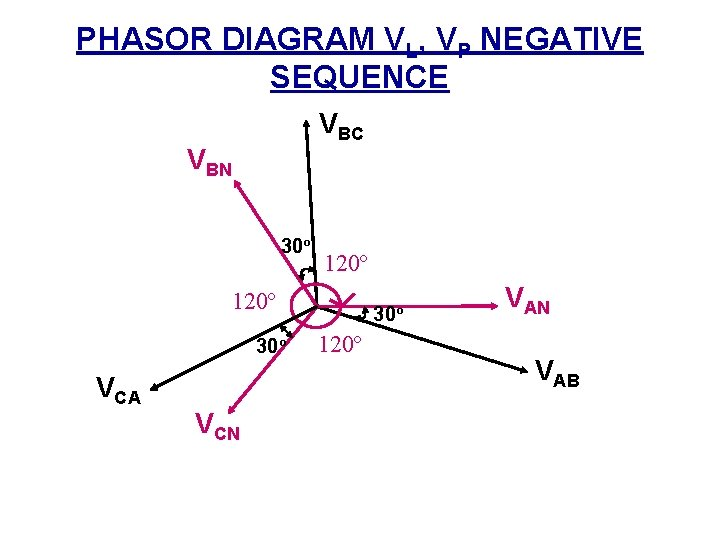PHASOR DIAGRAM VL, VP NEGATIVE SEQUENCE VBC VBN 30º 120º 30º VCA VCN 30º 120º VAN VAB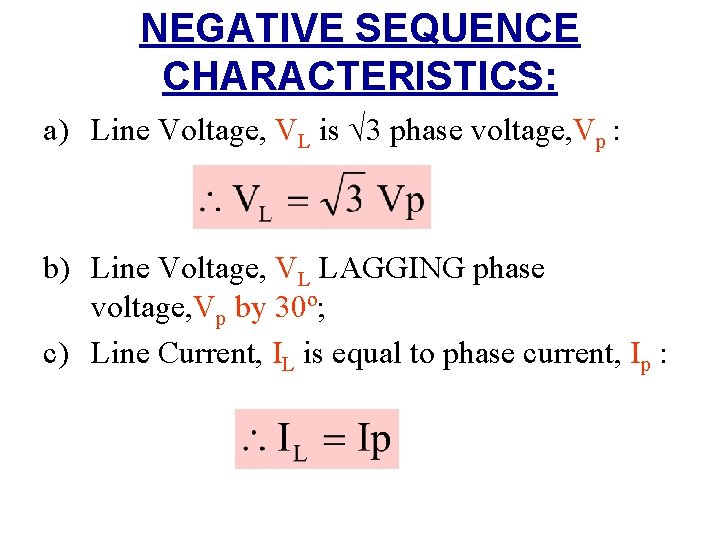NEGATIVE SEQUENCE CHARACTERISTICS: a) Line Voltage, VL is √ 3 phase voltage, Vp : b) Line Voltage, VL LAGGING phase voltage, Vp by 30º; c) Line Current, IL is equal to phase current, Ip :(2) DELTA-CONNECTION OR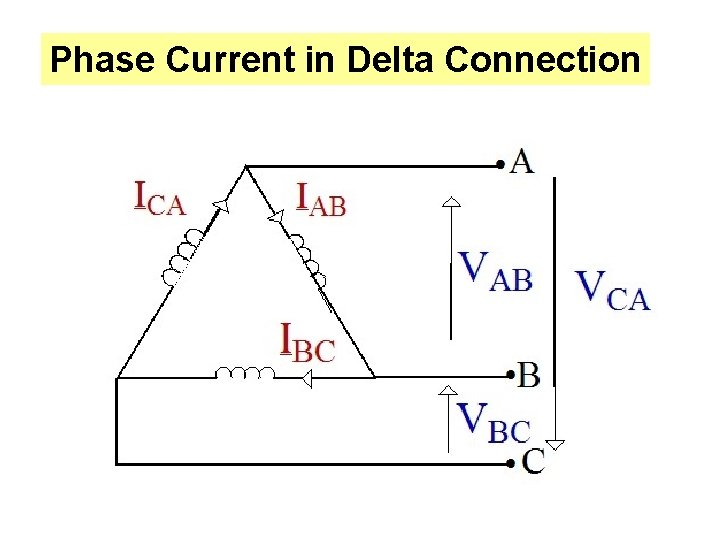Phase Current in Delta ConnectionLine Current in Delta Connection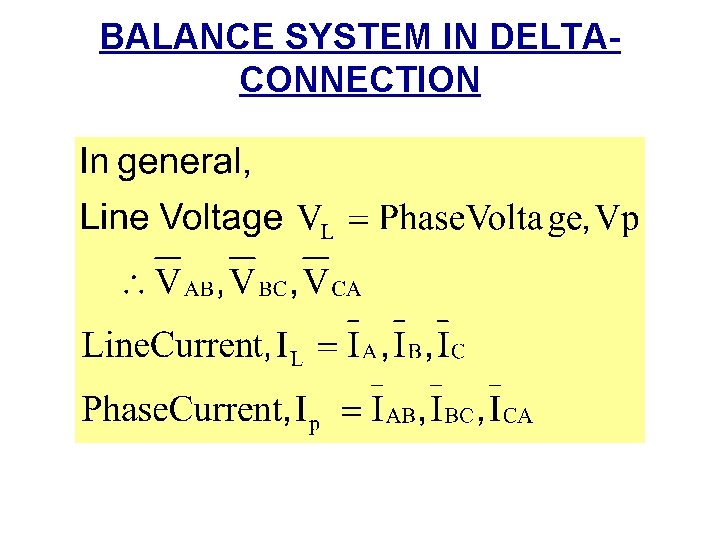BALANCE SYSTEM IN DELTACONNECTIONRELATIONSHIP BETWEEN PHASE CURRENT AND LINE CURRENT a) POSITIVE SEQUENCE, ABC b) NEGATIVE SEQUENCE, ACBLine Current/Voltage in Delta Connection(a) POSITIVE SEQUENCE By considered phase current IAB as a reference;Line Current A can be accomplished as follow:Line Current B can be accomplished as follow: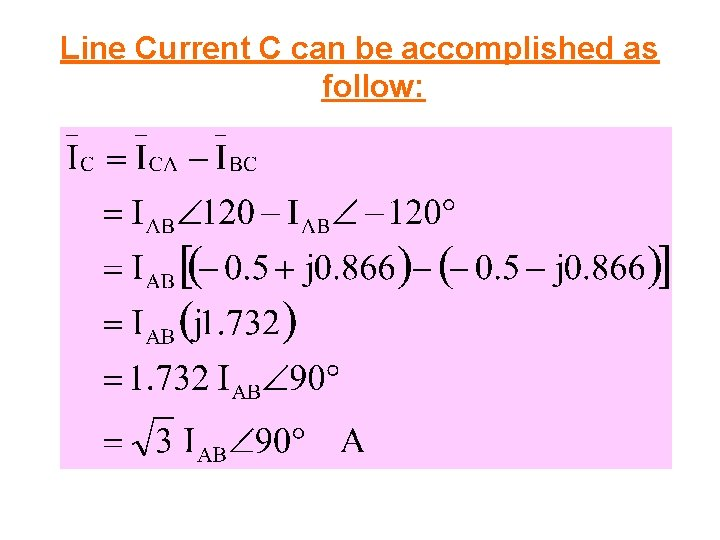Line Current C can be accomplished as follow: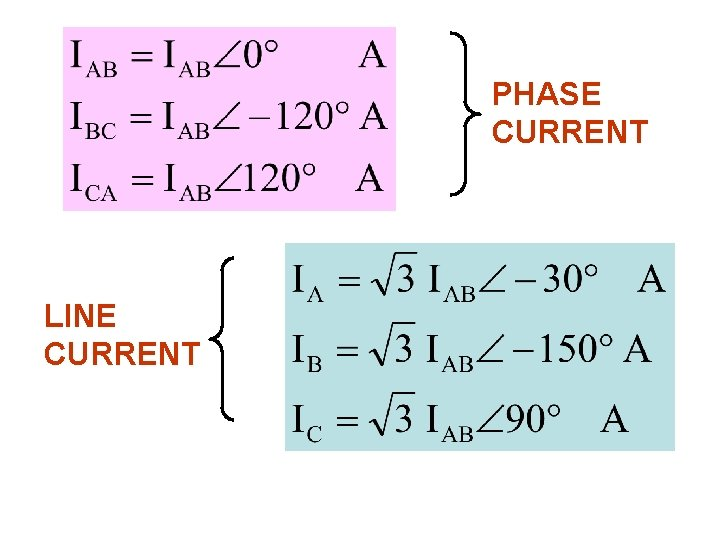PHASE CURRENT LINE CURRENT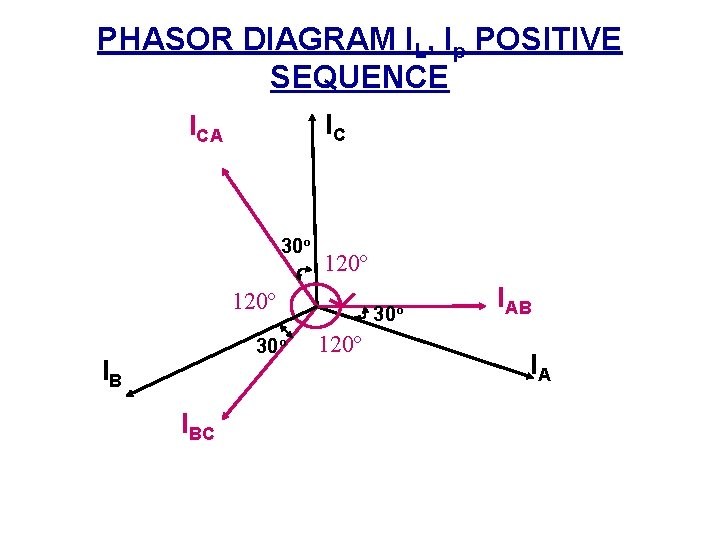PHASOR DIAGRAM IL, Ip POSITIVE SEQUENCE IC ICA 30º 120º 30º IB IBC 30º 120º IAB IAPOSITIVE SEQUENCE CHARACTERISTICS: a) Line current, IL is √ 3 phase current, Ip : b) Line current, IL LAGGING phase current, Ip by 30º; c) Line Voltage, VL is equal to phase voltage, Vp :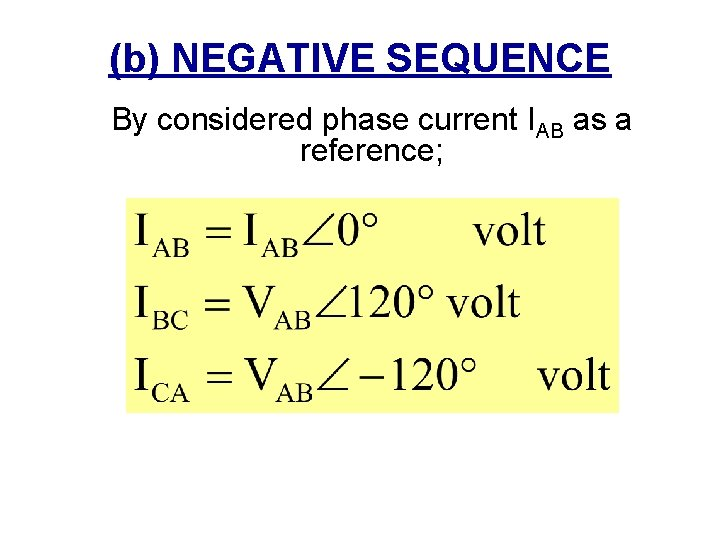(b) NEGATIVE SEQUENCE By considered phase current IAB as a reference;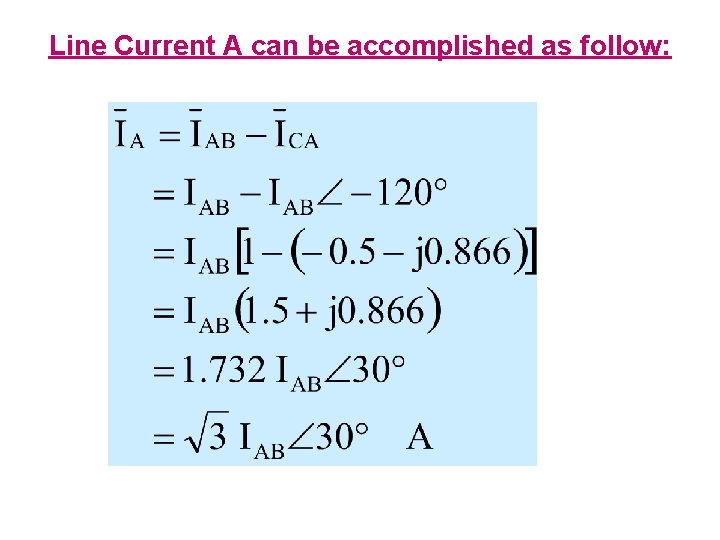Line Current A can be accomplished as follow: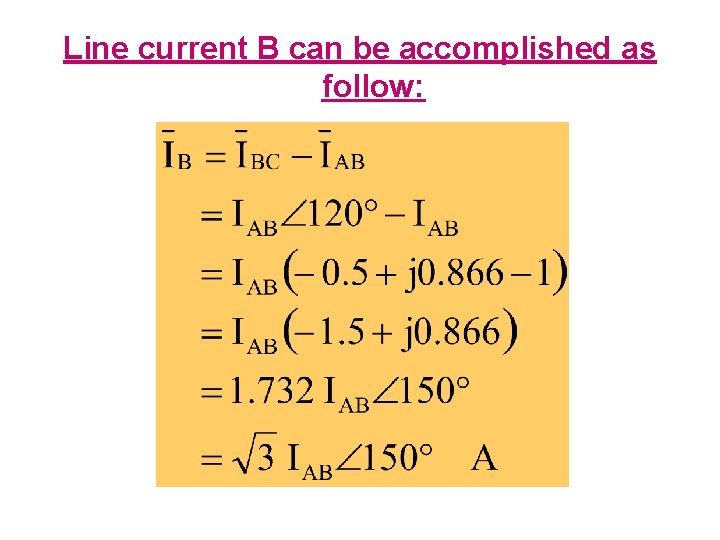Line current B can be accomplished as follow:Line Current C can be accomplished as follow:PHASE CURRENT LINE CURRENT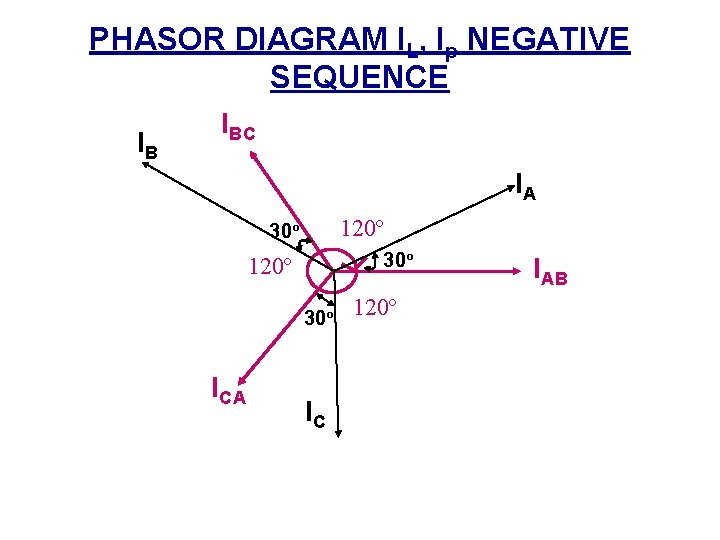PHASOR DIAGRAM IL, Ip NEGATIVE SEQUENCE IB IBC IA 120º 30º ICA IC 120º IAB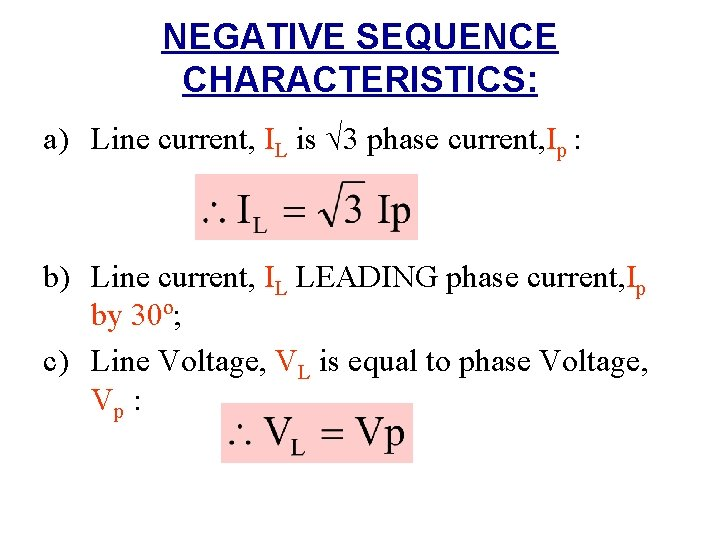NEGATIVE SEQUENCE CHARACTERISTICS: a) Line current, IL is √ 3 phase current, Ip : b) Line current, IL LEADING phase current, Ip by 30º; c) Line Voltage, VL is equal to phase Voltage, Vp :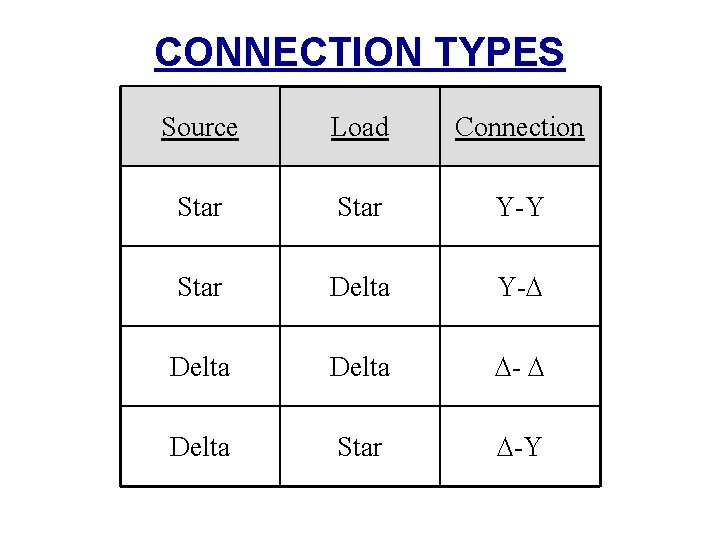CONNECTION TYPES Source Load Connection Star Y-Y Star Delta Y- Delta Star -YExample 1 A balanced three phase Y-connection generator with positive sequence has an impedance of 1. 2+j 0. 5Ω per phase and inner voltage 240 V per phase. The generator connected with load balanced three-phase Y-connection that has an impedance of 38+j 24Ω per phase. Line Impedance that connected to the source and load is 1. 8+j 2. 5Ω per phase. If inner voltage of a-phase generator is considered as reference,a) Define the a-phase equivalent circuit for this system. b) Calculate the line current Ia. A, Ib. B a and Ic. C c) Calculate all the three phase voltage at the load, VAN, VBN and VCN d) Calculate all the three line voltage, VAB, VBC and VCA at the load terminal. e) Calculate the phase voltage at generator/source terminal, Van, Vbn and Vcn f) Calculate the line voltage Vab, Vbc and Vca at the generator/source terminal.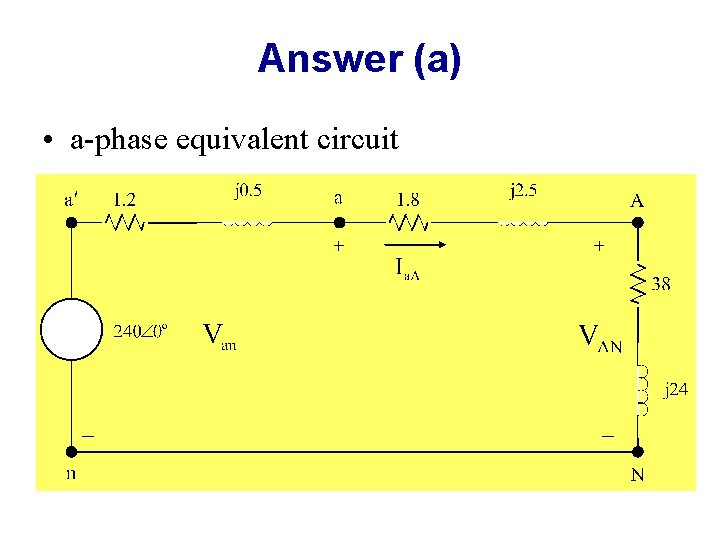Answer (a) • a-phase equivalent circuit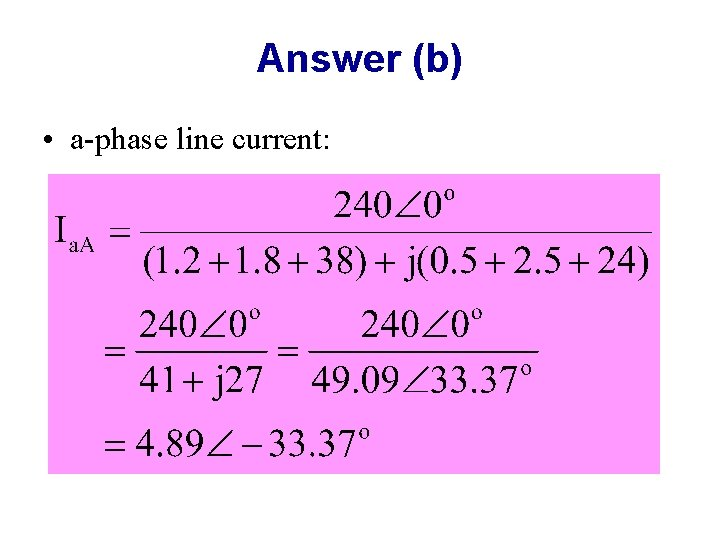Answer (b) • a-phase line current:• For the positive sequence: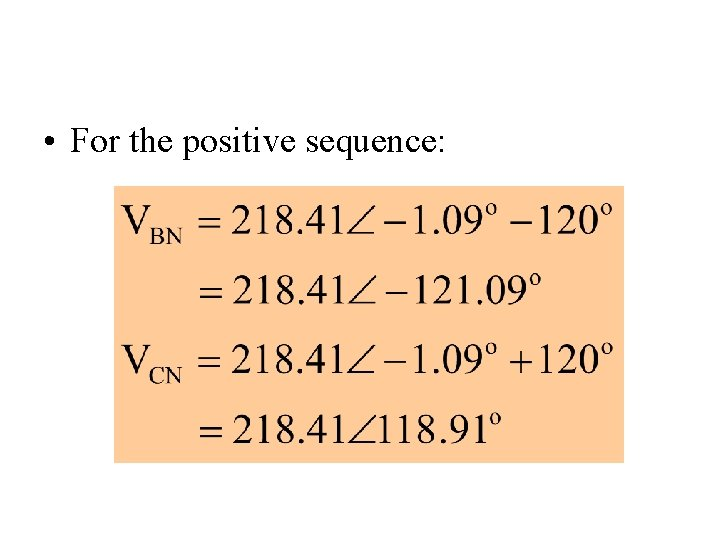• For the positive sequence: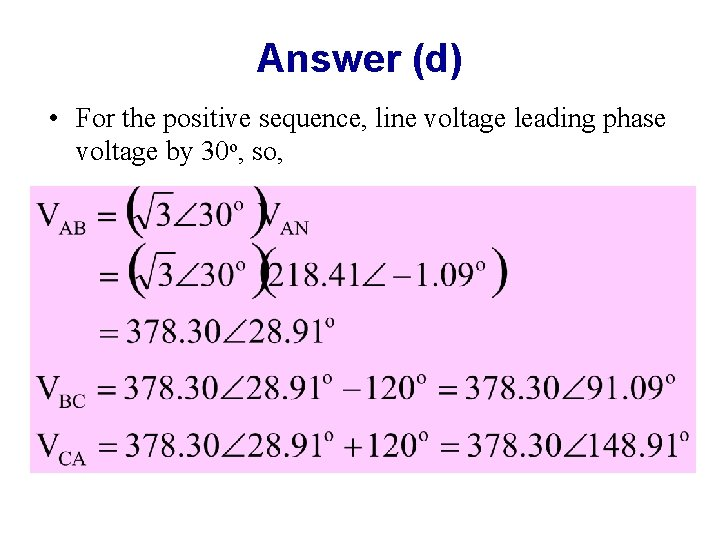Answer (d) • For the positive sequence, line voltage leading phase voltage by 30 o, so,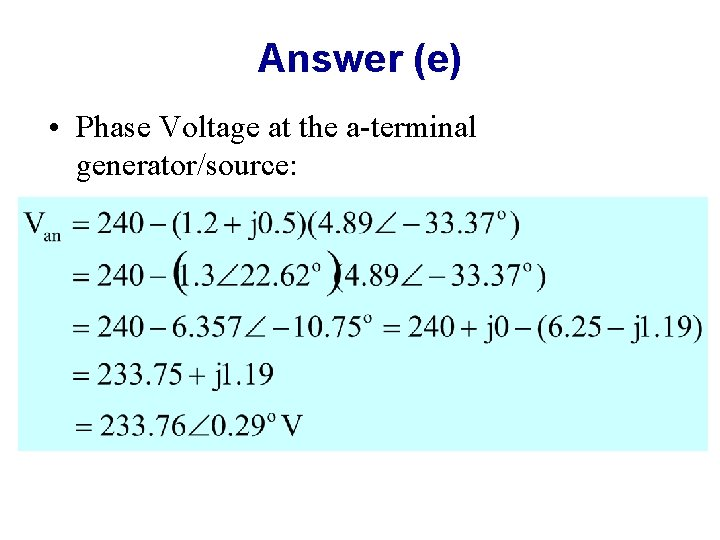Answer (e) • Phase Voltage at the a-terminal generator/source: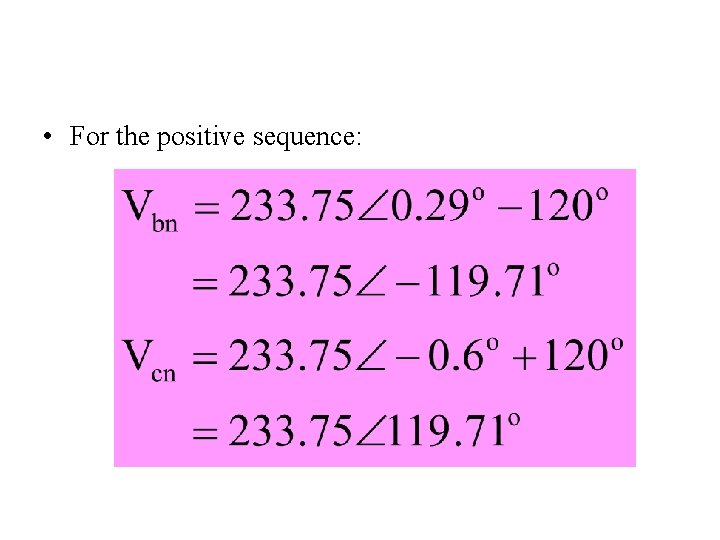• For the positive sequence:Answer (f) • Line Voltage at the generator terminal:POWER CALCULATION • AVERAGE POWER IN BALANCED STAR LOAD • COMPLEX POWER IN BALANCED STAR LOAD • POWER CALCULATION IN BALANCED DELTA LOADSTAR-CONNECTED LOAD IA A + IB IC B C + VBN ZB ZA - N ZC VAN VBN +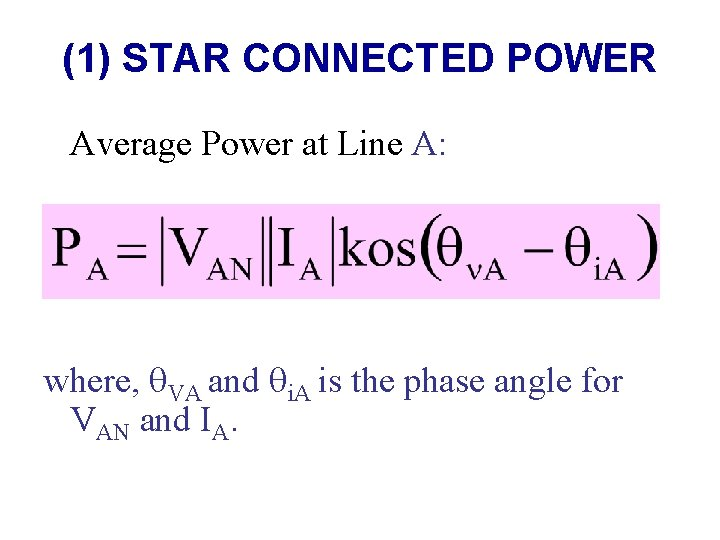(1) STAR CONNECTED POWER Average Power at Line A: where, VA and i. A is the phase angle for VAN and IA.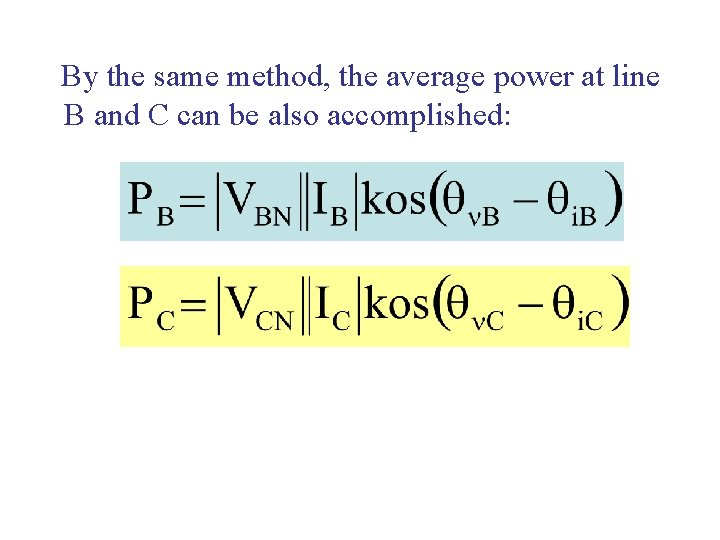By the same method, the average power at line B and C can be also accomplished: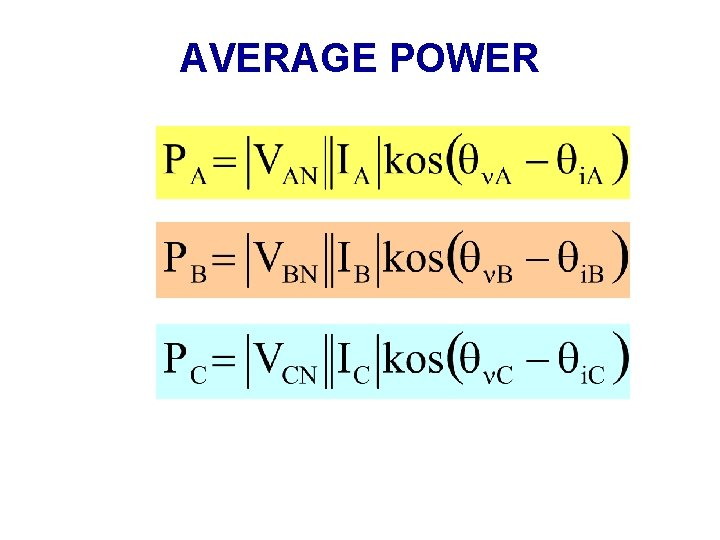AVERAGE POWERIn balanced star-connected three-phase system, MAGNITUDE for each phase-line voltage and phase current is EQUAL: Phase-line Voltage Phase-line current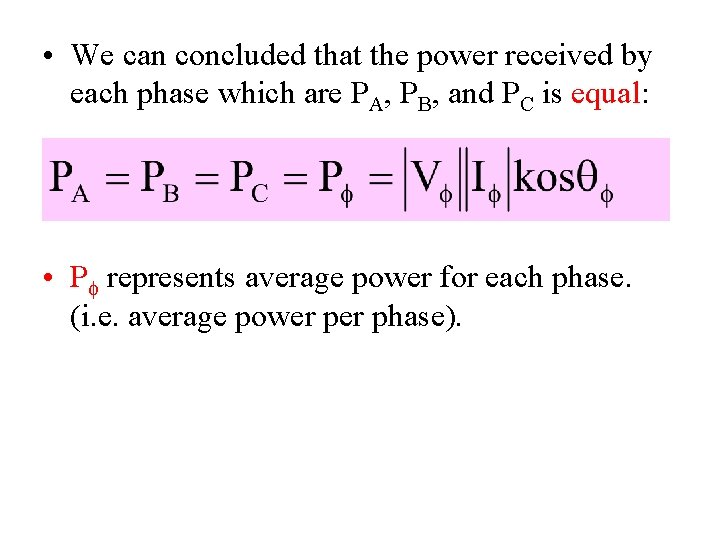• We can concluded that the power received by each phase which are PA, PB, and PC is equal: • P represents average power for each phase. (i. e. average power phase).• By knowing TOTAL average power that been received by load in balanced three-phase system, we just total up the average power for each phase: • PT represents the total average power that been received by the load in star-connected three-phase system.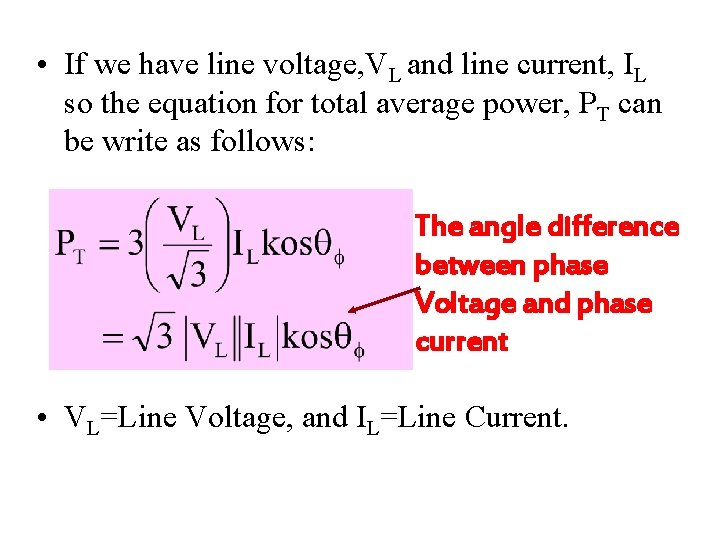• If we have line voltage, VL and line current, IL so the equation for total average power, PT can be write as follows: The angle difference between phase Voltage and phase current • VL=Line Voltage, and IL=Line Current.REMEMBER! • Phase Voltage normally can be represented by Vp or V. • Phase Voltage for star-connection are as follows: VAN, VBN, and VCN.TOTAL AVERAGE POWER, PT Total average power in phase voltage and phase current. Total average power in line voltage and line current.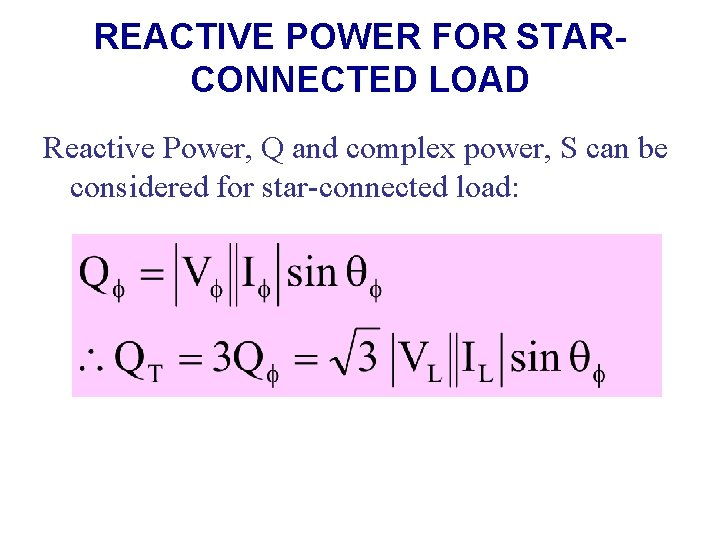REACTIVE POWER FOR STARCONNECTED LOAD Reactive Power, Q and complex power, S can be considered for star-connected load:COMPLEX POWER • Complex Power can be calculated as follows: • where, V and I represents voltage and current for the specific phase. So, generally: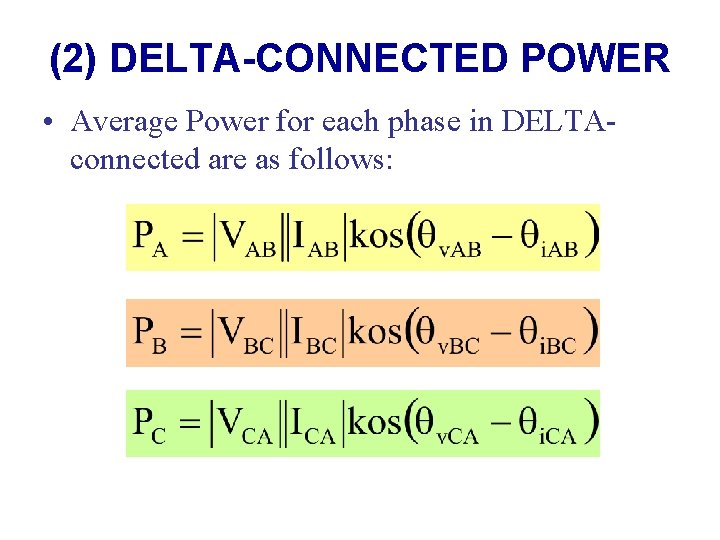(2) DELTA-CONNECTED POWER • Average Power for each phase in DELTAconnected are as follows: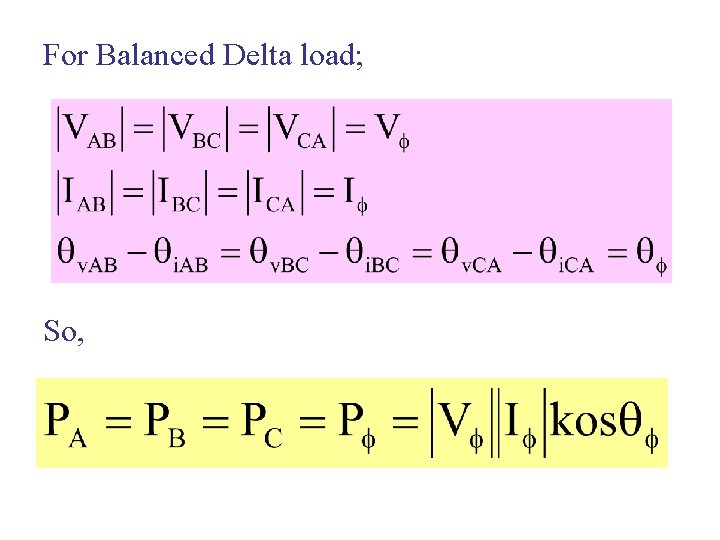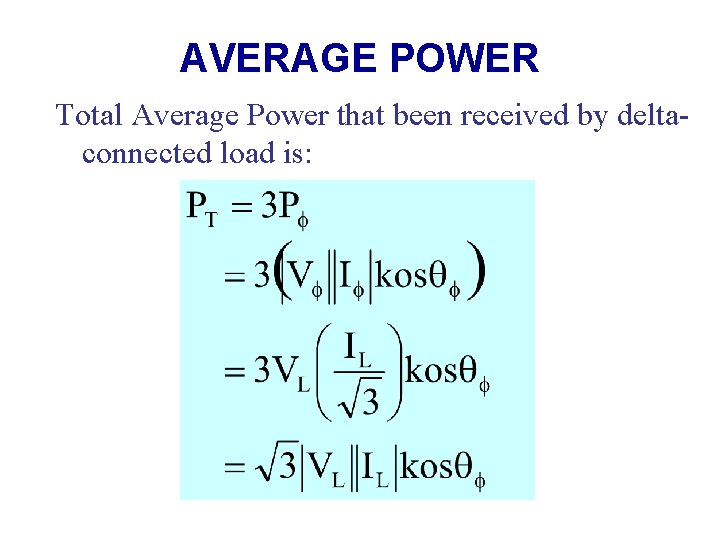AVERAGE POWER Total Average Power that been received by deltaconnected load is: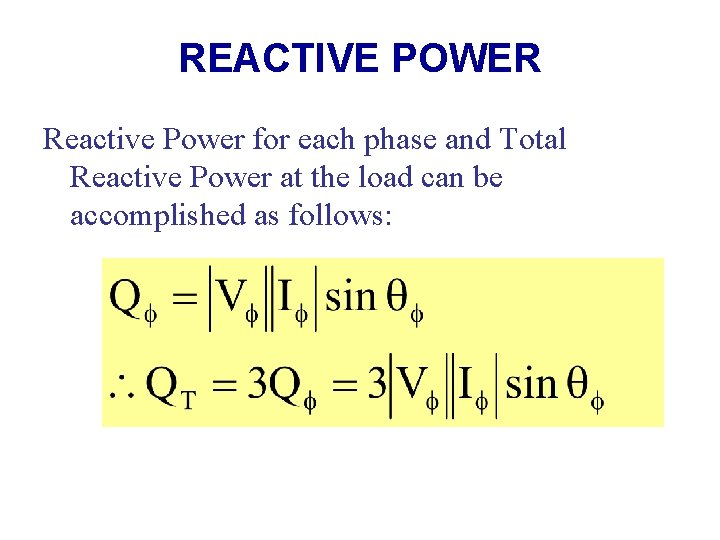REACTIVE POWER Reactive Power for each phase and Total Reactive Power at the load can be accomplished as follows: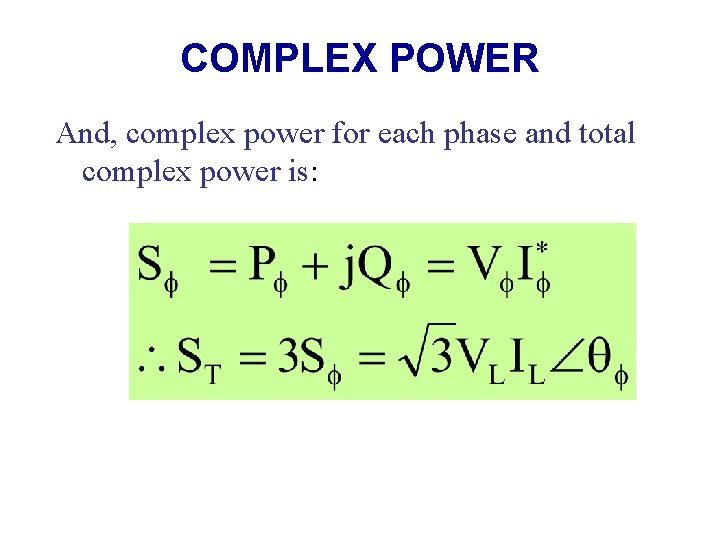COMPLEX POWER And, complex power for each phase and total complex power is: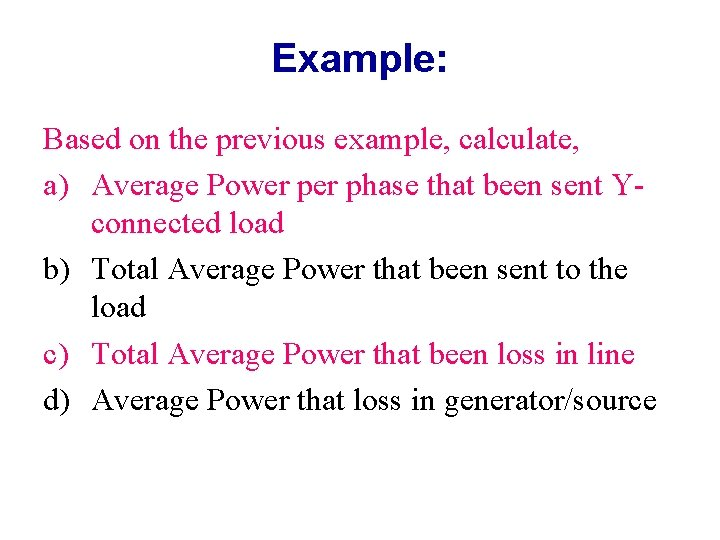Example: Based on the previous example, calculate, a) Average Power phase that been sent Yconnected load b) Total Average Power that been sent to the load c) Total Average Power that been loss in line d) Average Power that loss in generator/source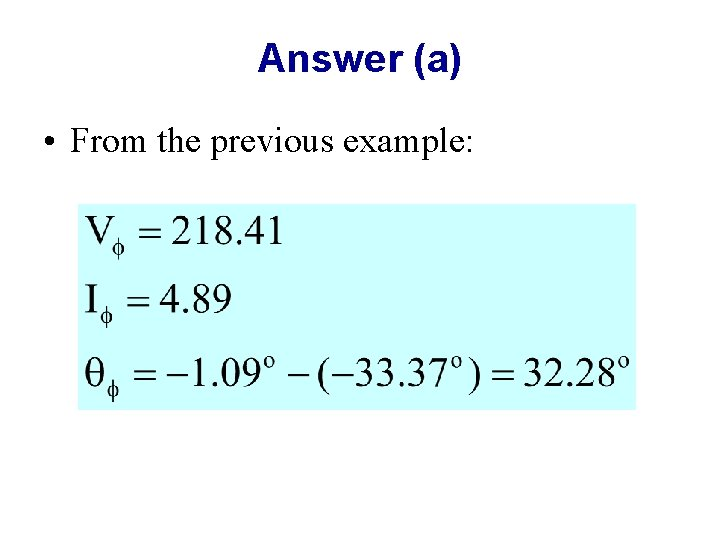Answer (a) • From the previous example: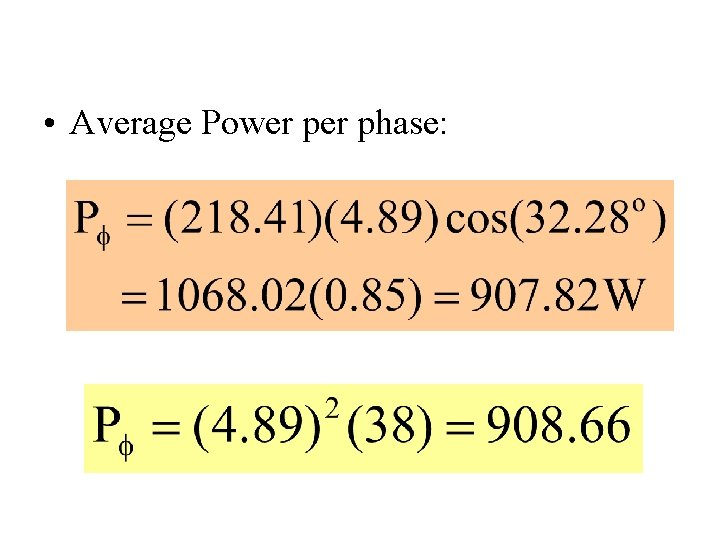• Average Power phase:Answer (b) • Total Average Power that been sent to the load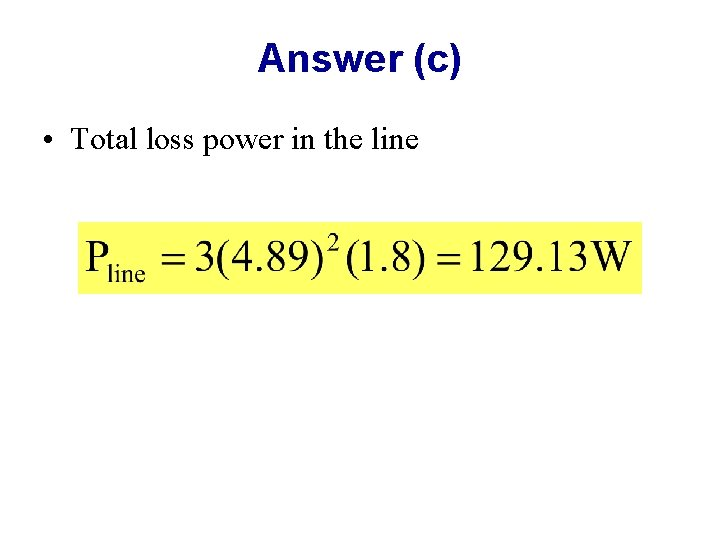Answer (c) • Total loss power in the line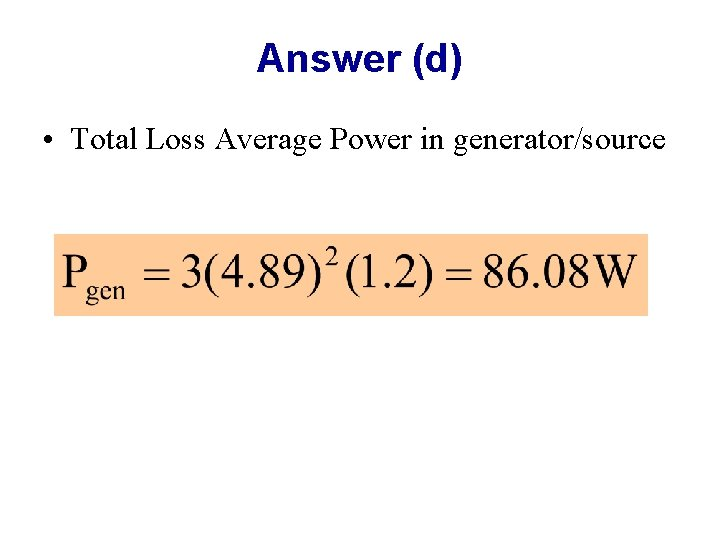Answer (d) • Total Loss Average Power in generator/source